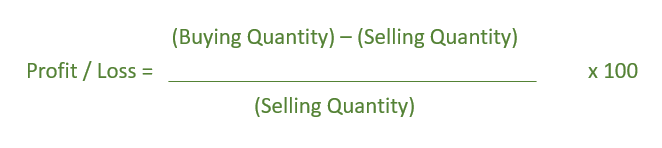# Program to find the profit or loss when CP of N items is equal to SP of M items

Givenanddenoting that the Cost Price of N articles is equal to the Selling Price of M articles. The task is to determine the profit or Loss percentage.

Examples:

```Input:  N = 8, M = 9
Output: Loss = -11.11%

Input:  N = 8, M = 5
Output: Profit = 60%
```

## Recommended: Please try your approach on {IDE} first, before moving on to the solution.

Formula:-Below is the implementation of the above approach:

## C++

 `// C++ implementation of above formula ` `#include ` `using` `namespace` `std; ` ` `  `// Function to calculate ` `// Profit or loss ` `void` `profitLoss(``int` `N, ``int` `M) ` `{ ` `    ``if` `(N == M) ` `        ``cout << ``"No Profit nor Loss"``; ` `    ``else` `{ ` ` `  `        ``float` `result = 0.0; ` ` `  `        ``result = ``float``(``abs``(N - M)) / M; ` ` `  `        ``if` `(N - M < 0) ` `            ``cout << ``"Loss = -"` `<< result * 100 << ``"%"``; ` `        ``else` `            ``cout << ``"Profit = "` `<< result * 100 << ``"%"``; ` `    ``} ` `} ` ` `  `// Driver Code ` `int` `main() ` `{ ` `    ``int` `N = 8, M = 9; ` ` `  `    ``profitLoss(N, M); ` ` `  `    ``return` `0; ` `} `

## Java

 `// Java implementation of above formula ` `public` `class` `GFG{ ` ` `  `    ``// Function to calculate ` `    ``// Profit or loss ` `    ``static` `void` `profitLoss(``int` `N, ``int` `M) ` `    ``{ ` `        ``if` `(N == M) ` `            ``System.out.print(``"No Profit nor Loss"``); ` `        ``else` `{ ` `     `  `            ``float` `result = ``0``; ` `     `  `            ``result = (``float``)(Math.abs(N - M))/ M; ` `     `  `            ``if` `(N - M < ``0``) ` `                ``System.out.print(``"Loss = -"` `+ result * ``100` `+``"%"``); ` `            ``else` `                ``System.out.print(``"Profit = "` `+ result * ``100` `+ ``"%"``); ` `        ``} ` `    ``} ` `     `  `    ``// Driver Code ` `     ``public` `static` `void` `main(String []args){ ` `          `  `        ``int` `N = ``8``, M = ``9``; ` `     `  `        ``profitLoss(N, M); ` `         `  `     ``} ` `     ``// This code is contributed by ANKITRAI1 ` `} `

## Python3

 `# Python 3 implementation of above formula ` ` `  `# Function to calculate Profit or loss ` `def` `profitLoss(N, M): ` `    ``if` `(N ``=``=` `M): ` `        ``print``(``"No Profit nor Loss"``) ` `    ``else``: ` `        ``result ``=` `0.0` ` `  `        ``result ``=` `float``(``abs``(N ``-` `M)) ``/` `M ` ` `  `        ``if` `(N ``-` `M < ``0``): ` `            ``print``(``"Loss = -"``,``'{0:.6}'` `.  ` `                   ``format``(result ``*` `100``), ``"%"``) ` `        ``else``: ` `            ``print``(``"Profit = "``,``'{0:.6}'` `.  ` `                   ``format``(result ``*` `100``), ``"%"``) ` ` `  `# Driver Code ` `if` `__name__ ``=``=` `'__main__'``: ` `    ``N ``=` `8` `    ``M ``=` `9` ` `  `    ``profitLoss(N, M) ` ` `  `# This code is contributed by ` `# Sanjit_Prasad `

## C#

 `// C# implementation of above formula  ` `using` `System; ` ` `  `class` `GFG ` `{ ` `// Function to calculate  ` `// Profit or loss  ` `static` `void` `profitLoss(``int` `N, ``int` `M)  ` `{  ` `    ``if` `(N == M)  ` `        ``Console.Write(``"No Profit nor Loss"``);  ` `    ``else` `    ``{  ` `        ``float` `result = 0;  ` ` `  `        ``result = (``float``)(Math.Abs(N - M))/ M;  ` ` `  `        ``if` `(N - M < 0)  ` `            ``Console.Write(``"Loss = -"` `+  ` `                           ``result * 100 +``"%"``);  ` `        ``else` `            ``Console.Write(``"Profit = "` `+  ` `                           ``result * 100 + ``"%"``);  ` `    ``}  ` `}  ` ` `  `// Driver Code  ` `static` `public` `void` `Main () ` `{ ` `    ``int` `N = 8, M = 9;  ` ` `  `    ``profitLoss(N, M);  ` `}  ` `} ` ` `  `// This code is contributed by ajit.  `

## PHP

 ` `

Output:

```Loss = -11.1111%
```

Don’t stop now and take your learning to the next level. Learn all the important concepts of Data Structures and Algorithms with the help of the most trusted course: DSA Self Paced. Become industry ready at a student-friendly price.

My Personal Notes arrow_drop_upCheck out this Author's contributed articles.

If you like GeeksforGeeks and would like to contribute, you can also write an article using contribute.geeksforgeeks.org or mail your article to contribute@geeksforgeeks.org. See your article appearing on the GeeksforGeeks main page and help other Geeks.

Please Improve this article if you find anything incorrect by clicking on the "Improve Article" button below.